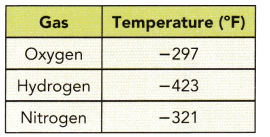# Math in Focus Grade 7 Chapter 2 Lesson 2.2 Answer Key Subtracting Integers

Go through the Math in Focus Grade 7 Workbook Answer Key Chapter 2 Lesson 2.2 Subtracting Integers to finish your assignments.

## Math in Focus Grade 7 Course 2 A Chapter 2 Lesson 2.2 Answer Key Subtracting Integers

### Math in Focus Grade 7 Chapter 2 Lesson 2.2 Guided Practice Answer Key

Hands-On Activity

Materials:

• counters

Subtract Integers

Work in pairs.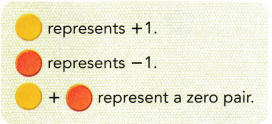A zero pair can be added to any expression without changing its value. It is the same thing as adding 0 to a number.
Sometimes, you add zero pairs in order to subtract.

Step 1.
Use counters to model and complete the subtraction of a positive integer.

a) Evaluate 5 – (+2) and compare with 5 + (-2).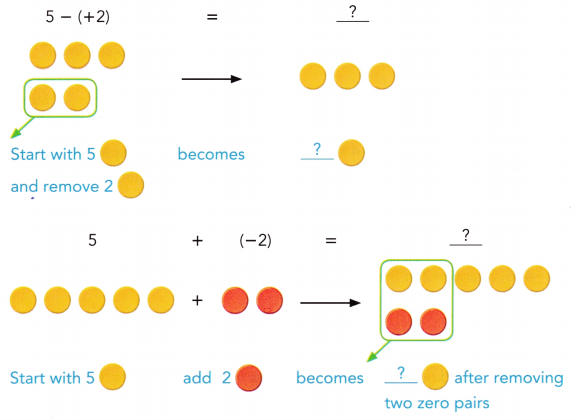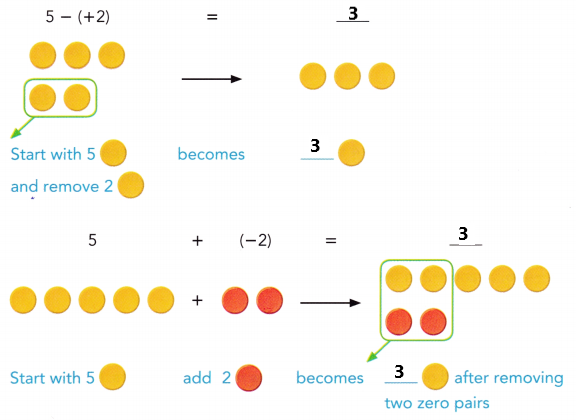b) Evaluate -5 – (+2) and compare with -5 + (-2).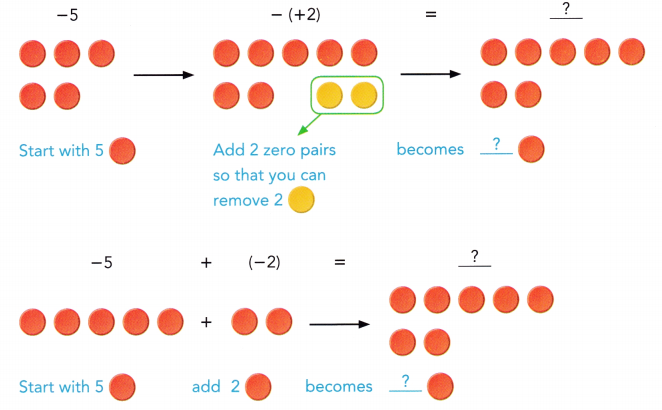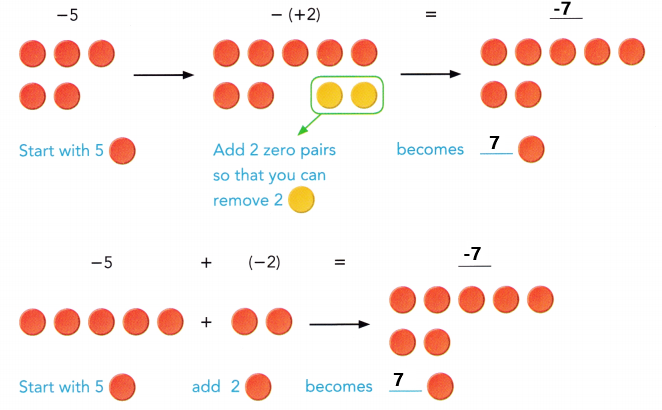Step 2
Use counters to evaluate each expression.
a) 6 – 4 and 6 + (-4)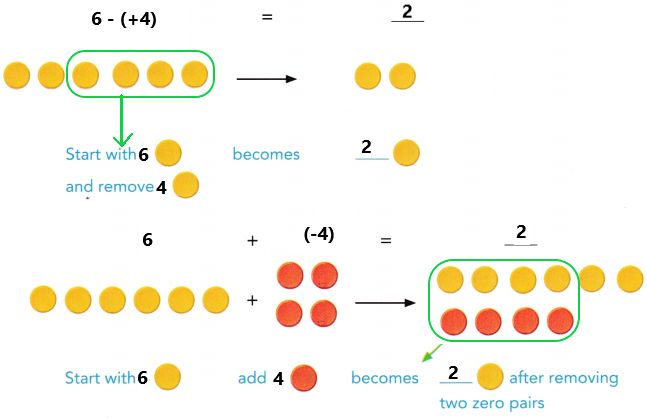b) -6 – 4 and -6 + (-4)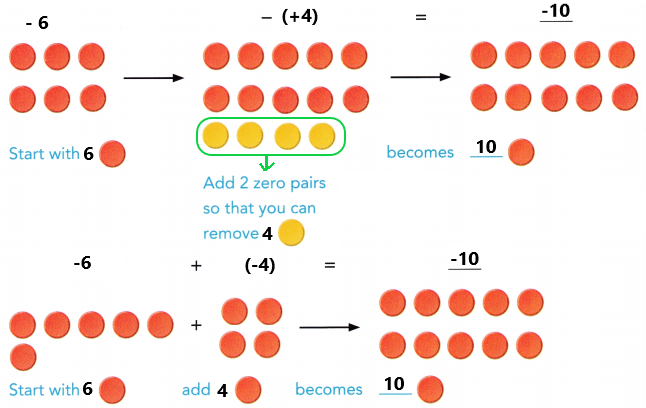Step 3
Use counters to model and complete the subtraction of a negative integer,

a) Evaluate 5 – (-2) and compare with 5 + 2.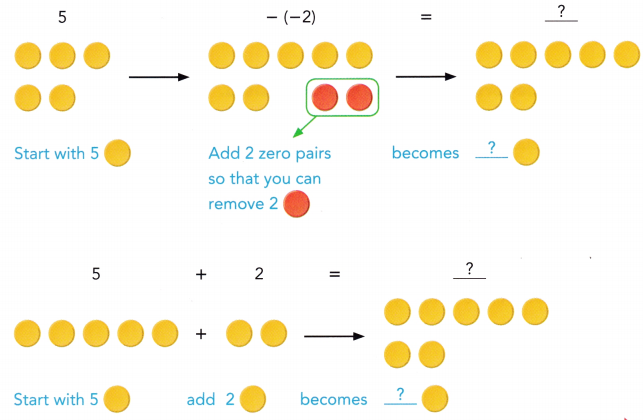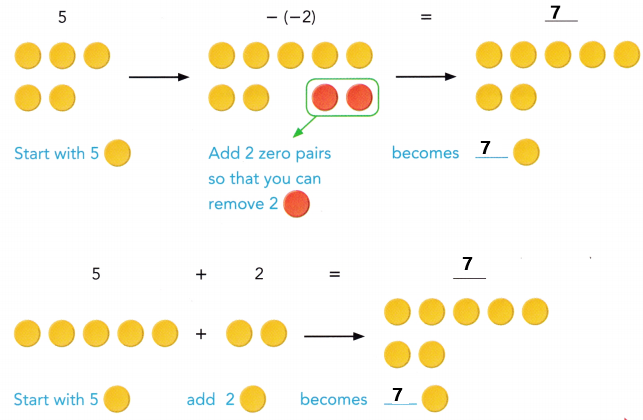b) Find -5 – (-2) and compare with -5 + 2.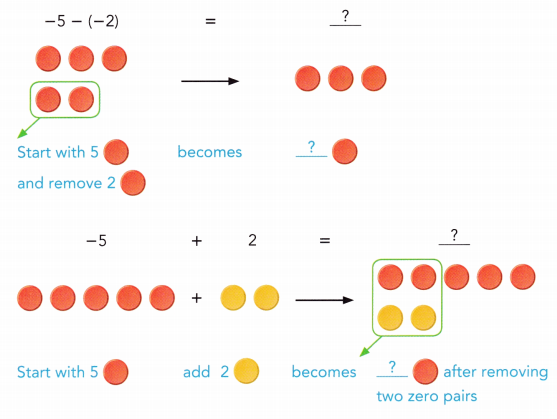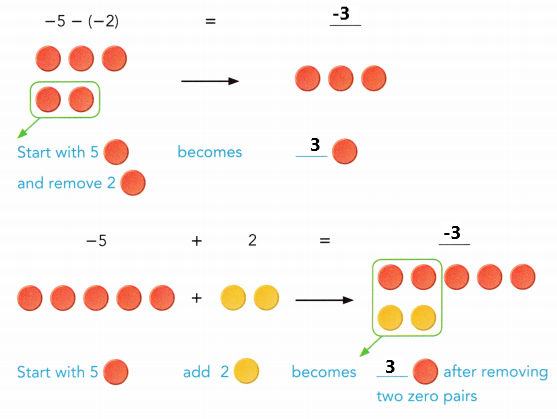Step 4
Use counters to evaluate each expression.

a) 7 – (-3) and 7 + 3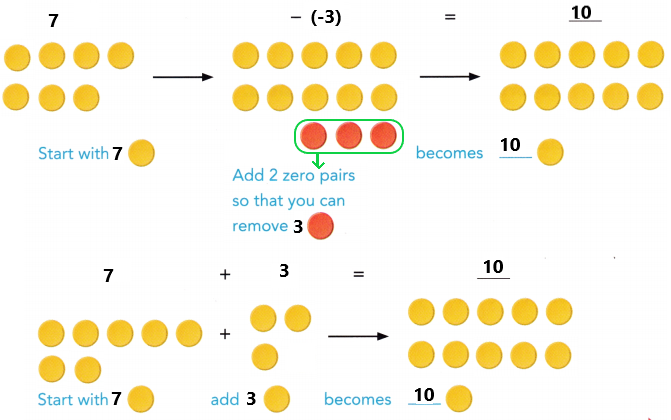b) -7 – (-3) and -7 + 3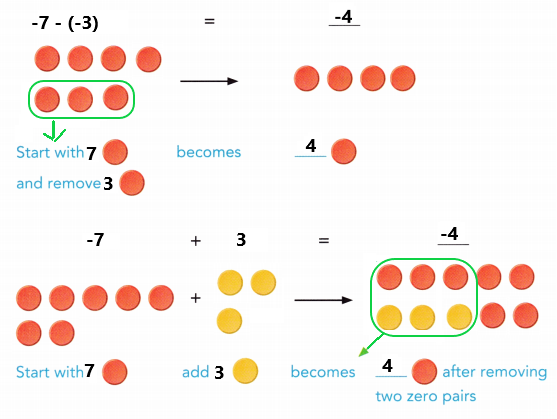Math Journal Based on your results in step 1 to step 4, explain how you can subtract integers.

Copy and complete.

Question 1.
21 – 30
21 – 30 = 21 +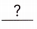Rewrite subtraction as adding the opposite.
Using absolute values,
|-30| – |21| = 30 – 21 Subtract the absolute values, because the addends have different signs.
=Simplify.
21 – 30 = 21 +=Use a negative sign, because -30 has a greater absolute value.
=Rewrite subtraction as adding the opposite.
21 – 30 = 21 + (-30)
Using absolute values,
|-30| – |21| = 30 – 21
Subtract the absolute values, because the addends have different signs.
= 9
21 – 30 = 21 + (-30)
= -9 Use a negative sign, because -30 has a greater absolute value.
= -9

Question 2.
A fishing boat drags its net 35 feet below the ocean’s surface. Then it lowers the net by an additional 12 feet. Find the new position relative to the surface of the fishing net.
-35 –= -35 +Rewrite subtraction as adding the opposite.
Using absolute values,+=Add the absolute values because the addends have the same sign.
-35 –= -35 +Use the common sign, a negative sign, for the sum.
=Simplify.
The fishing net’s new position isfeet below the surface of the ocean.
Rewrite subtraction as adding the opposite.
-35 – 12 = -35 + (-12)
Using absolute values,
|-35||-12| = 47
-35 – 12 = -35 + (-12)
Use the common sign, a negative sign, for the sum.
= -47
The fishing net’s new position is 47 feet below the surface of the ocean.

Solve.

Question 3.
A submarine was at 1,200 feet below sea level, It then moved to 1,683 feet below sea level. How many feet did the submarine descend?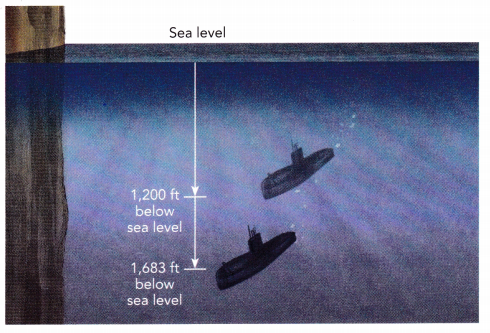1,683 – 1,200 = 493 feet
The submarine descend to 493 feet below sea level.
Explanation:
A submarine was at 1,200 feet below sea level, It then moved to 1,683 feet below sea level. Subtract 1,200 feet from 1,683 feet the difference is 493 feet. The submarine descend to 493 feet below sea level.

Copy and complete.

Question 4.
17 – (-4)
17 – (-4) = 17 +Rewrite subtraction as adding the opposite.
=Add.
17 – (-4)
Rewrite subtraction as adding the opposite.
= 17 +
= 21
Explanation:
Perform subtraction operation one above numbers. Rewrite subtraction as adding the opposite. Add 17 with4 the sum is 21.

Evaluate each expression.

Question 5.
-25 – (-9)
-25 – (-9)
Rewrite subtraction as adding the opposite.
= -25 + 9
= -16
Explanation:
Perform subtraction operation one above numbers. Rewrite subtraction as adding the opposite. Add -25 with 9 the sum is -16.

Question 6.
-19 – (-7) – (-6)
-19 – (-7) – (-6)
= -19 + 7 + 6
= -19 + 13
= -6
Explanation:
Perform subtraction operation one above numbers. Rewrite subtraction as adding the opposite. Add 7 with 6 the sum is 13. Next add -19 with 13 the sum is -6.

Copy and complete.

Question 7.
Find the distance between 3 and -2.
Method 1
Use a number line to plot the points and count the units.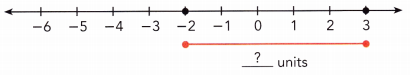The distance between 3 and -2 isunits.
Method 2
Use absolute value to find the distance between integers with opposite signs.
Distance between 3 and -2:
|3 –| = || Rewrite subtraction as adding the opposite.
=units Add.
The distance between 3 and -2 isunits.
Use a number line to plot the points and count the units.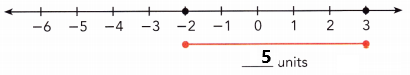The distance between 3 and -2 is 5 units.
Method 2
Use absolute value to find the distance between integers with opposite signs.
Distance between 3 and -2:
Rewrite subtraction as adding the opposite.
|3 – (-2) | = | 3 + 2 |
= 5 units
The distance between 3 and -2 is units.

Question 8.
A particular town has an elevation of 8 feet below sea level. Another town on top of a mountain has an elevation of 2,421 feet above sea level. What is the difference in the elevations of the two towns?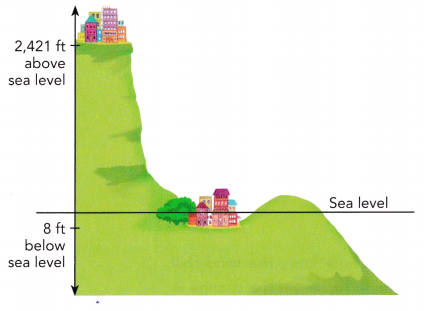Elevation of town below sea level:ft
Elevation of town on top of mountain:ft
Difference between the two elevations:
|| = || Rewrite subtraction as adding the opposite.
=ft Add.
The difference in the elevations of the two towns isfeet.
Elevation of town below sea level: -8 feet
Elevation of town on top of mountain: 2,421 feet
Difference between the two elevations:
Rewrite subtraction as adding the opposite.
| 2,421(-8) | = | 2,421 + 8 |
= 2,429 feet
The difference in the elevations of the two towns is 2,429 feet.

### Math in Focus Course 2A Practice 2.2 Answer Key

Evaluate each expression.

Question 1.
7 – 18
7 – 18 = -11
Explanation:
Perform subtraction operation on above two numbers. Subtract 18 from 7 the difference is -11.

Question 2.
20 – 30
20 – 30 = -10
Explanation:
Perform subtraction operation on above two numbers. Subtract 30 from 20 the difference is -10.

Question 3.
53 – 109
53 – 109 = -56
Explanation:
Perform subtraction operation on above two numbers. Subtract 109 from 53 the difference is -56.

Question 4.
45 – (-16)
45 – (-16)
= 45 + 16
= 61
Explanation:
Perform subtraction operation on above two numbers. Rewrite subtraction as adding the opposite. Add 45 with 16 the sum is 61.

Question 5.
-7 – (-5)
-7 – (-5)
= -7 + 5
= -2
Explanation:
Perform subtraction operation on above two numbers. Rewrite subtraction as adding the opposite. Add -7 with 5 the sum is -2.

Question 6.
-94 – (-68)
-94 – (-68)
= -94 + 68
= -26
Explanation:
Perform subtraction operation on above two numbers. Rewrite subtraction as adding the opposite. Add -94 with 68 the sum is -26.

Question 7.
-6 – 8 – 12
-6 – 8 – 12
= -14 – 12
= -26
Explanation:
Perform addition operation on above numbers. Add -6 with -8 the sum is -14. Add -14 with -12 the sum is -26.

Question 8.
-23 – 17 – 7
-23 – 17 – 7
= -40 – 7
= -47
Explanation:
Perform addition operation on above numbers. Add -23 with -17 the sum is -40. Add -40 with -7 the sum is -47.

Question 9.
-8 – (-4) – 5
-8 – (-4) – 5
= – 8 + 4 -5
= -13 + 4
= -9
Explanation:
Perform subtraction operation on above numbers. Rewrite subtraction as adding the opposite. Add -8 with -5 the sum is -13. Add -13 with 4 the sum is -9.

Question 10.
-5 – (-10) – 6
-5 – (-10) – 6
= – 5 + 10 – 6
= 5 – 6
= -1
Explanation:
Perform subtraction operation on above numbers. Rewrite subtraction as adding the opposite. Add -5 with 10 the sum is 5. Add 5 with -6 the sum is -1.

Question 11.
-20 – (-16) – (-7)
-20 – (-16) – (-7)
= -20 + 16 + 7
= -20 + 23
= 3
Explanation:
Perform subtraction operation on above numbers. Rewrite subtraction as adding the opposite. Add 16 with 7 the sum is 23. Add -20 with 23 the sum is 3.

Question 12.
-11 – (-8) – (-14)
-11 – (-8) – (-14)
= -11 + 8 + 14
= -11 + 22
= 11
Explanation:
Perform subtraction operation on above numbers. Rewrite subtraction as adding the opposite. Add 8 with 14 the sum is 22. Add -11 with 22 the sum is 11.

Evaluate the distance between each pair of integers.

Question 13.
4 and 20
Use a number line to plot the points and count the units.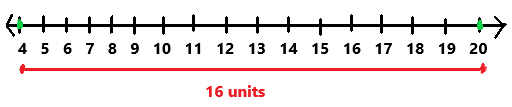The distance between 4 and 20 is 16 units.

Question 14.
16 and 52
52 – 16 = 36
The distance between 16 and 52 is 36.

Question 15.
-15 and 36
Use absolute value to find the distance between integers with opposite signs.
Distance between -15 and 36:
Rewrite subtraction as adding the opposite.
|-15 + 36 | = | 15 + 36 |
= 51 units
The distance between -15 and 36 is 51 units.

Question 16.
-7 and 41
Use absolute value to find the distance between integers with opposite signs.
Distance between -7 and 41:
Rewrite subtraction as adding the opposite.
|-7 + 41 | = | 7 + 41|
= 48 units
The distance between -7 and 41 is 48 units.

Question 17.
-28 and -3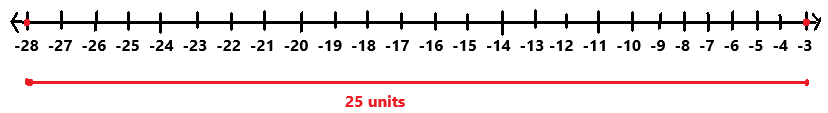The distance between -28 and -3 is 25 units.

Question 18.
-19 and -8
Use a number line to plot the points and count the units.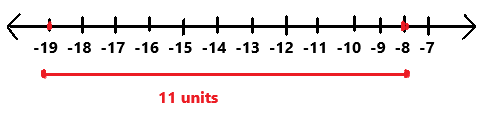The distance between -19 and -8 is 11 units.

Question 19.
Rick leaves to go skiing in Burlington, Vermont, when the temperature is -4°C. The temperature drops 10°C when a cold front moves in. What is the new temperature?
-4°C – 10°C = -14°C
The new temperature is -14°C.
Explanation:
When the temperature is -4°C Rick leaves to go skiing in Burlington, Vermont. The temperature drops 10°C when a cold front moves in. Which is represented as -10°C. Add -4°C with -10°C the sum is -14°C. The new temperature is -14°C.

Question 20.
The water level of the Dead Sea dropped from 390 meters below sea level in 1930 to 423 meters below sea level in 2010. By how much did the water level drop from 1930 to 2010?
423 – 390 = 33 meters
The water level drop 33 meters below sea level from 1930 to 2010.
Explanation:
The water level of the Dead Sea dropped from 390 meters below sea level in 1930 to 423 meters below sea level in 2010. Subtract 390 meters from 423 meters the difference is 33 meters.

Question 21.
Math Journal Florence has only $420 in her bank account. Describe how to find the amount in her account after she writes a check for$590.
$420 –$590 = -$170 Explanation: Florence has only$420 in her bank account. Then she writes a check of $590. Subtract$590 from $420 the difference is -$170. The amount in her account is -\$170.

Question 22.
Math Journal Darren has trouble simplifying 15 – (-36). Write an explanation to help him.
15 – (-36)
= 15 + 36
= 51
Explanation:
Perform subtraction operation on above numbers. Rewrite subtraction as adding the opposite. Add 15 with 36 the sum is 51.

Question 23.
The wind-chill temperature at 10 P.M. was -8°F. One hour later, the wind-chill temperature had fallen to -28°F. Write an expression to represent the change in temperature. Then find the change in temperature.
(-28) – (-8)
= -28 + 8
= -20°F
The change in temperature is -20°F.
Explanation:
The wind-chill temperature at 10 P.M. was -8°F. One hour later, the wind-chill temperature had fallen to -28°F. The expression to represent the change in temperature is -(28) – (-8). After solving the expression the change in temperature is -20°F.

Question 24.
The lowest point in North America is in Death Valley, California, which is 86 meters below sea level at its lowest point. The highest point is Denali, a mountain in Alaska, with an elevation of 6,198 meters above sea level. What is the difference in their elevations?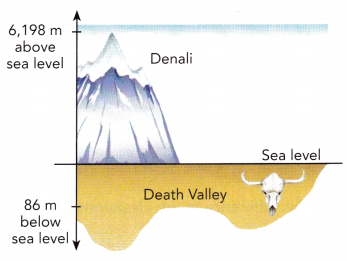6,198 m – (-86m)
= 6,198 m + 86 m
= 6,284 m
The difference in their elevations is 6,284 meters.
Explanation:
The lowest point in North America is in Death Valley, California, which is 86 meters below sea level at its lowest point. It is represented as -86 meters. The highest point is Denali, a mountain in Alaska, with an elevation of 6,198 meters above sea level. The difference in their elevations is 6,284 m.

Question 25.
Belinda has two freezers. Freezer A keeps frozen foods at a temperature of -20°F, while Freezer B keeps frozen foods at a temperature of -4°F. She transferred a package of frozen food from one freezer to the other.
a) What is the temperature difference between the two freezers?
-4°F – (-20°F)
= -4°F + 20°F
= 16°F
The temperature difference between the two freezers are 16°F.

b) If the temperature of the package rises after the transfer, from which freezer was the package taken?
The package was taken from freezer A. Freezer A keeps frozen foods at a temperature of -20°F, while Freezer B keeps frozen foods at a temperature of -4°F. The temperature -4°F is greater than -20°F. The temperature of the package rises after the transfer.

Question 26.
You and a friend are playing a video game. Your score so far is 340 points and your friend’s score is -220 points. What is the difference between your scores?
340 – (-220)
= 340 + 220
= 560
The difference between our scores is 560 points.

Question 27.
Two record low monthly temperatures for Anchorage, Alaska, are -34°F in January and 31°F in August. Find the difference between these two temperatures.
31°F – (-34°F)
= 31°F + 34°F
= 65°F
The difference between the two temperatures is 65°F.
Explanation:
Two record low monthly temperatures for Anchorage, Alaska, are -34°F in January and 31°F in August. Add 31°F with 34°F the sum is 65°F. The difference between the two temperatures is 65°F.

Question 28.
Town X is 120 feet above sea level, Town Y is 25 feet below sea level, and Town Z is 30 feet below sea level. How high is
a) Town X above Town Y?
Town X is 120 feet above sea level. Above sea level is represented with +120 feet.
Town Y is 25 feet below sea level. Below sea level is represented with -25 feet.
-25 feet + 120 feet = 95 feet.
Town X is 95 feet higher than Town Y.

b) Town Y above Town Z? Town X
Town Y is 25 feet below sea level. Below sea level is represented with -25 feet.
Town Z is 30 feet above sea level. Below sea level is represented with -30 feet.
-25 feet – (-30) feet = 5 feet.
Town Y is 5 feet higher than Town Z.

c) Town X above Town Z?
Town X is 120 feet above sea level. Above sea level is represented with +120 feet.
Town Z is 30 feet below sea level. Below sea level is represented with -30 feet.
-30 feet + 120 feet = 90 feet.
Town X is 90 feet higher than Town Z.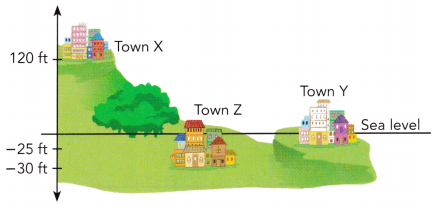Question 29.
Math Journal
a) Find |8 – 12| and |8| – |12|. Is |8 – 12| equal to |8| – |12|?
|8 – 12|
= |-4|
= 4
|8| – |12|
= 8 – 12
= -4
No, |8 – 12| is not equal to |8| – |12|

b) Find |12 – 8| and |12| – |8|. Is |12 – 8| equal to |12| – |8|?
|12 – 8|
= |4|
= 4
|12| – |8|
= 12 – 8
= 4
Yes, |12 – 8| equal to |12| – |8|

c) Joe thinks that to find the distance between two integers m and n, he can write |m| – |n| or |n| – |m|. Use your answer in a) and b) to explain why you agree or disagree.